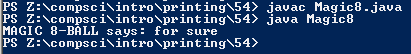# Assignment #54 and Magic 8-Ball

## Code

```       /// Name: Mahima Choudhary
/// Period: 5
/// Program Name: Magic 8-Ball
/// File Name: Magic8.java
/// Date Finihsed: 11/9/2015

import java.util.Random;

public class Magic8 {

public static void main ( String[] args ) {

Random r = new Random();

int choice = 1 + r.nextInt(20);
String response = "";

if ( choice == 1 )
response = "This is the truth";
else if ( choice == 2 )
response = "No doubt";
else if ( choice == 3 )
response = "Indubitobly";
else if ( choice == 4 )
response = "If thats what your heart desires";
else if ( choice == 5 )
response = "absolutely";
else if ( choice == 6 )
response = "You can count on that";
else if ( choice == 7 )
response = "Very much so";
else if ( choice == 8 )
response = "Lifes looking preety good for you";
else if ( choice == 9 )
response = "for sure";
else if ( choice == 10 )
response = "Yes";
else if ( choice == 11 )
response = "im not in the mood";
else if ( choice == 12 )
response = "Maybe Later";
else if ( choice == 13 )
response = "TTYL";
else if ( choice == 14 )
response = "Im hungry";
else if ( choice == 15 )
response = "Are you there?";
else if ( choice == 16 )
response = "i dont think so";
else if ( choice == 17 )
response = "sorry, no";
else if ( choice == 18 )
response = "uh most likely not";
else if ( choice == 19 )
response = "ive got bad news";
else if ( choice == 20 )
response = "no";
else
response = "8-BALL is confused, ask again...or dont!";

System.out.println( "MAGIC 8-BALL says: " + response );
}
}
```

### Picture of the output